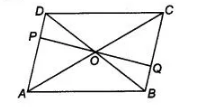# P and O are points on opposite sides AD and BC`
Question:

P and O are points on opposite sides AD and BC of a parallelogram ABCD such that PQ passes through the point of intersection O of its diagonals AC

and BD. Show that PQ is bisected at O.

Thinking Process

Firstly, prove that ΔODP and ΔOBQ are congruent by ASA rule. Further show the required result by CPCT rule

Solution:

Given $A B C D$ is a parallelogram whose diagonals bisect each other at $O$. To show $P Q$ is bisected at $O$.In $\triangle O D P$ and $\triangle O B Q$,

$\angle B O Q=\angle P O D$ [since, vertically opposite angles]

$\angle O B Q=\angle O D P$ [ alternate interior angles]

and $\quad O B=O D \quad$ [given]

$\begin{array}{lll}\therefore & \Delta O D P \cong \Delta O B Q & \text { [by ASA congruence rule ] }\end{array}$

$\therefore \quad O P=O Q$ [by CPCT rule]

So, $P Q$ is bisected at $O$.

Hence proved.